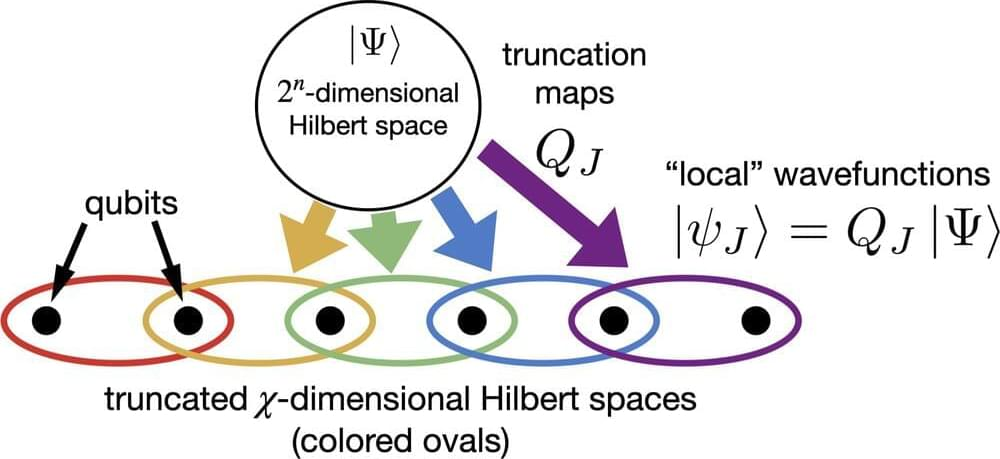Kevin Slagle, Quantum 7, 1113 (2023). Although tensor networks are powerful tools for simulating low-dimensional quantum physics, tensor network algorithms are very computationally costly in higher spatial dimensions. We introduce $\textit{quantum gauge networks}$: a different kind of tensor network ansatz for which the computation cost of simulations does not explicitly increase for larger spatial dimensions. We take inspiration from the gauge picture of quantum dynamics, which consists of a local wavefunction for each patch of space, with neighboring patches related by unitary connections. A quantum gauge network (QGN) has a similar structure, except the Hilbert space dimensions of the local wavefunctions and connections are truncated. We describe how a QGN can be obtained from a generic wavefunction or matrix product state (MPS). All $2k$-point correlation functions of any wavefunction for $M$ many operators can be encoded exactly by a QGN with bond dimension $O(M^k)$. In comparison, for just $k=1$, an exponentially larger bond dimension of $2^{M/6}$ is generically required for an MPS of qubits. We provide a simple QGN algorithm for approximate simulations of quantum dynamics in any spatial dimension. The approximate dynamics can achieve exact energy conservation for time-independent Hamiltonians, and spatial symmetries can also be maintained exactly. We benchmark the algorithm by simulating the quantum quench of fermionic Hamiltonians in up to three spatial dimensions.